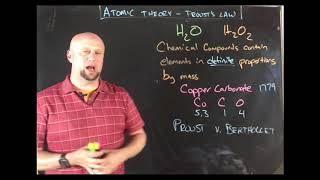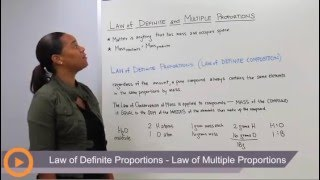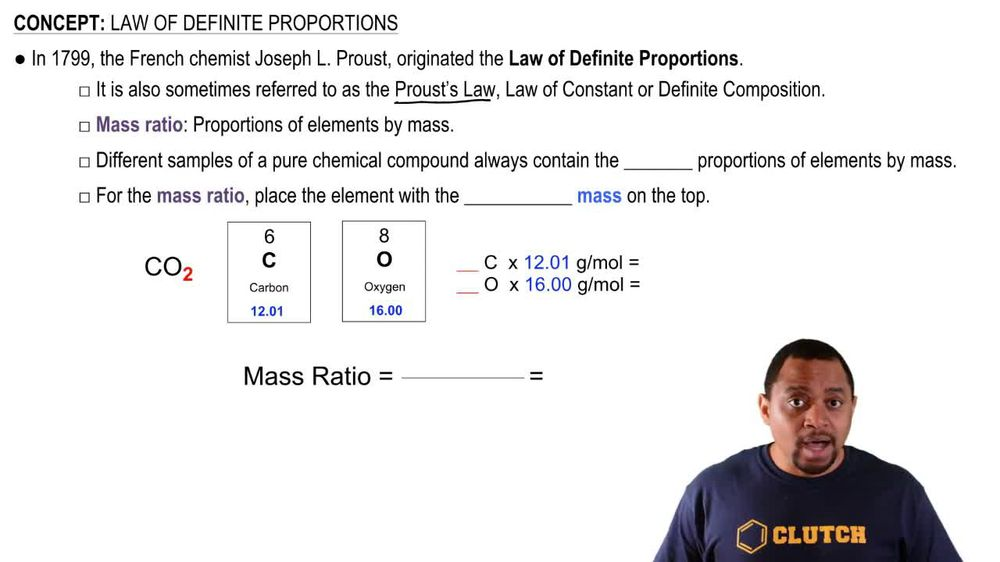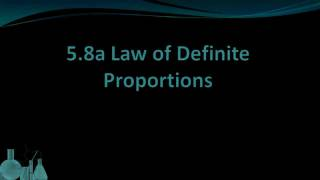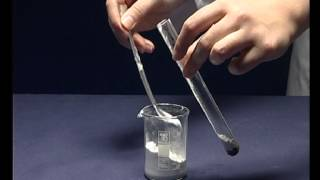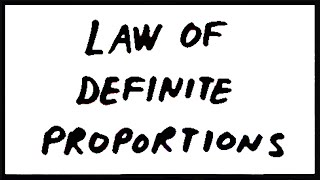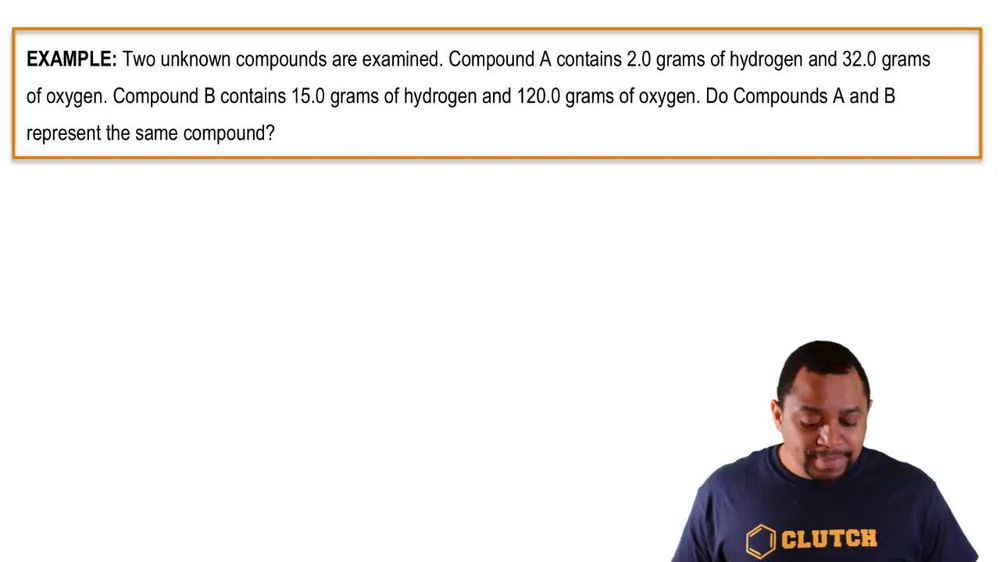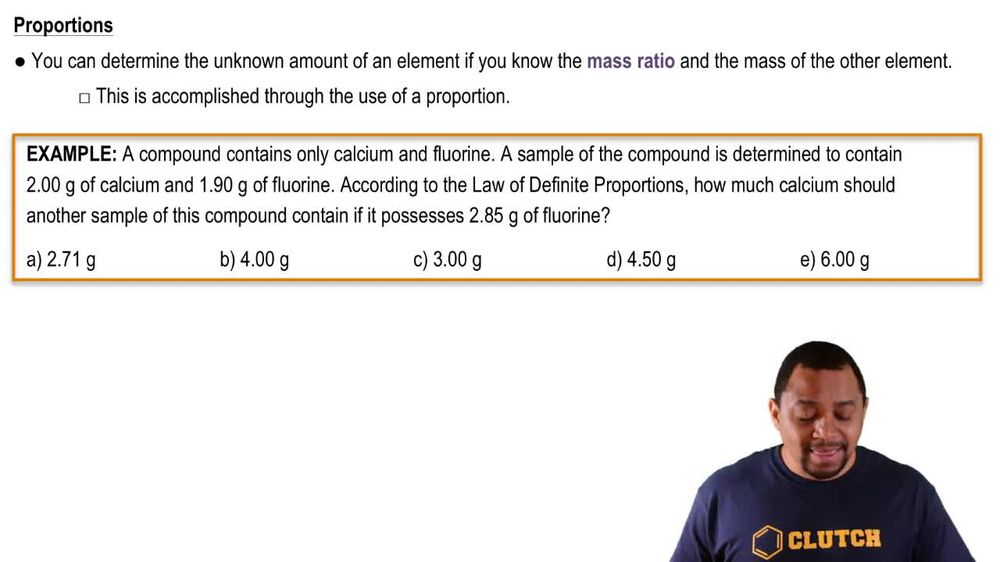Start typing, then use the up and down arrows to select an option from the list.
1. 2. Atoms & Elements2. Law of Definite Proportions
Problem

# A 1.0-g sample of carbon dioxide (CO2) is fully decomposed into its elements, yielding 0.273 g of carbon and 0.727 g of oxygen. (a) What is the ratio of the mass of O to C?

Relevant Solution1m
Play a video:
Hey everyone in this example, we need to determine the mass of chlorine per gram of carbon. For a compound made of 0.55 g 550 g of carbon and 6.45 g of chlorine. And so what this means is we want to recall our formula for the mass ratio, which is going to be taking our mass of our designated adam, which in this case is going to be our mass of chlorine. And dividing that by our mass given for carbon. And so what we're going to have is in our numerator are mass for chlorine given in the prompt as 6.45 g of chlorine and in the denominator are massive carbon given in the prompt as .550 g of carbon. And this is going to give us a value equal to 11.7. Well we'll still have our units of grams and this is going to be 11.7 g of chlorine per gram of carbon. And this will complete this example as our final answer. So I hope that everything I went through is clear. But if you have any questions, please leave them down below and I will see everyone in the next practice video